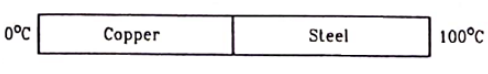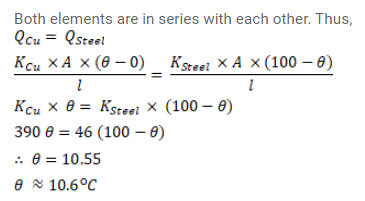# Figure shows a copper rod joined to a steel rod.Question:

Figure shows a copper rod joined to a steel rod. The rods have equal length and equal cross - sectional area. The free end of the copper rod is kept at $0^{\circ} \mathrm{C}$ and that of the steel rod is kept at $100^{\circ} \mathrm{C}$ Find the temperature at the junction of the rods. Conductivity of copper $=390 \mathrm{~W} / \mathrm{m}-{ }^{\circ} \mathrm{C}$ and that of steel $=46 \mathrm{~W} / \mathrm{m}$ - ${ }^{\circ} \mathrm{C}$.Solution: